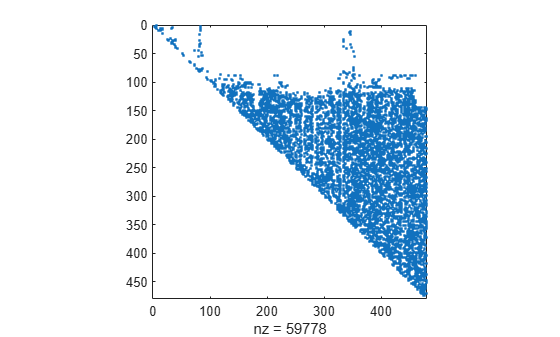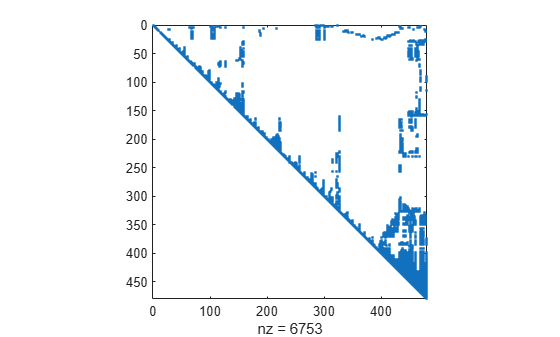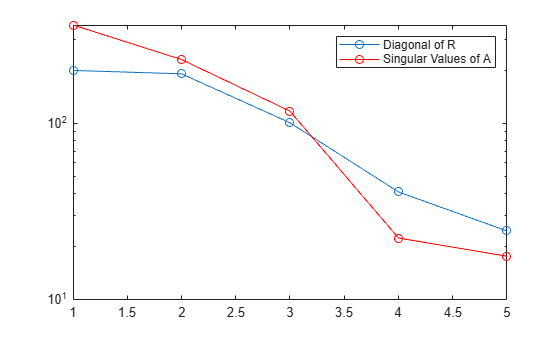# qr

QR decomposition

## Syntax

``R = qr(A)``
``[Q,R] = qr(A)``
``[Q,R,P] = qr(A)``
``[___] = qr(A,"econ")``
``[Q,R,P] = qr(A,outputForm)``
``[___] = qr(A,0)``
``[C,R] = qr(S,B)``
``[C,R,P] = qr(S,B)``
``[___] = qr(S,B,"econ")``
``[C,R,P] = qr(S,B,outputForm)``
``[___] = qr(S,B,0)``

## Description

example

````R = qr(A)` returns the upper-triangular `R` factor of the QR decomposition ```A = Q*R```.```

example

````[Q,R] = qr(A)` performs a QR decomposition on `m`-by-`n` matrix `A` such that `A = Q*R`. The factor `R` is an `m`-by-`n` upper-triangular matrix, and the factor `Q` is an `m`-by-`m` orthogonal matrix.```

example

````[Q,R,P] = qr(A)` additionally returns a permutation matrix `P` such that ```A*P = Q*R```. If `A` is full, the permutation matrix is chosen so that `abs(diag(R))` is decreasing.```

example

````[___] = qr(A,"econ")` produces an economy-size decomposition using any of the previous output argument combinations. The size of the outputs depends on the size of `m`-by-`n` matrix `A`: If `m > n`, then `qr` computes only the first `n` columns of `Q` and the first `n` rows of `R`.If `m <= n`, then the economy-size decomposition is the same as the regular decomposition. ```

example

````[Q,R,P] = qr(A,outputForm)` specifies whether to return the permutation information `P` as a matrix or a vector. For example, if `outputForm` is `"vector"`, then `A(:,P) = Q*R`. The default value of `outputForm` is `"matrix"` such that `A*P = Q*R`.```
````[___] = qr(A,0)` is equivalent to `qr(A,"econ","vector")`. The use of this syntax is not recommended. Use the `"econ"` option instead.```

example

````[C,R] = qr(S,B)` computes `C = Q'*B` and the upper-triangular factor `R`. You can use `C` and `R` to compute a least-squares solution to the sparse linear system `S*X = B` with ```X = R\C```.```

example

````[C,R,P] = qr(S,B)` additionally returns a permutation matrix `P` that is chosen to reduce fill-in in `R`. You can use `C`, `R`, and `P` to compute a least-squares solution to the sparse linear system `S*X = B` with `X = P*(R\C)`.```

example

````[___] = qr(S,B,"econ")` produces an economy-size decomposition using any of the previous output argument combinations. The size of the outputs depends on the size of `m`-by-`n` sparse matrix `S`: If `m > n`, then `qr` computes only the first `n` rows of `C` and `R`.If `m <= n`, then the economy-size decomposition is the same as the regular decomposition. ```

example

````[C,R,P] = qr(S,B,outputForm)` specifies whether to return the permutation information `P` as a matrix or vector. For example, if `outputForm` is `"vector"`, then the least-squares solution to `S*X = B` is ```X(P,:) = R\C```. The default value of `outputForm` is `"matrix"` such that the least-squares solution to ```S*X = B``` is `X = P*(R\C)`.```
````[___] = qr(S,B,0)` is equivalent to `qr(S,B,"econ","vector")`. The use of this syntax is not recommended. Use the `"econ"` option instead.```

## Examples

collapse all

Find the QR decomposition of the 5-by-5 magic square matrix. Specify one output argument to return just the upper-triangular factor.

```A = magic(5); R = qr(A)```
```R = 5×5 -32.4808 -26.6311 -21.3973 -23.7063 -25.8615 0 19.8943 12.3234 1.9439 4.0856 0 0 -24.3985 -11.6316 -3.7415 0 0 0 -20.0982 -9.9739 0 0 0 0 -16.0005 ```

Compute the full QR decomposition of a magic square test matrix by specifying two output arguments.

```A = magic(5); [Q,R] = qr(A)```
```Q = 5×5 -0.5234 0.5058 0.6735 -0.1215 -0.0441 -0.7081 -0.6966 -0.0177 0.0815 -0.0800 -0.1231 0.1367 -0.3558 -0.6307 -0.6646 -0.3079 0.1911 -0.4122 -0.4247 0.7200 -0.3387 0.4514 -0.4996 0.6328 -0.1774 ```
```R = 5×5 -32.4808 -26.6311 -21.3973 -23.7063 -25.8615 0 19.8943 12.3234 1.9439 4.0856 0 0 -24.3985 -11.6316 -3.7415 0 0 0 -20.0982 -9.9739 0 0 0 0 -16.0005 ```

Verify that $\mathit{A}=\mathrm{QR}$, within machine precision.

`norm(A-Q*R)`
```ans = 9.5562e-15 ```

Specify three output arguments to return a permutation matrix or vector that reduces fill-in in the `R` factor of the QR decomposition.

Compute the QR decomposition of the `west0479` sparse matrix. Specify three outputs to return a permutation matrix that satisfies $\mathrm{AP}=\mathrm{QR}$.

```load west0479 A = west0479; [Q,R,P] = qr(A);```

Verify that `A*P = Q*R` for the permutation matrix `P`, within machine precision.

`norm(A*P-Q*R,"fro")`
```ans = 3.3521e-10 ```

Now specify the `"vector"` option to return `p` as a permutation vector.

`[Q,R,p] = qr(A,"vector");`

Verify that `A(:,p) = Q*R` for the permutation vector `p`, within machine precision.

`norm(A(:,p) - Q*R,"fro")`
```ans = 3.3521e-10 ```

Verify that the use of a permutation matrix or permutation vector in the decomposition results in an `R` factor with fewer nonzeros for sparse inputs compared to a nonpermuted decomposition.

```[Q1,R1] = qr(A); spy(R1)````spy(R)`The results show that the permuted decomposition produces an `R` factor with substantially fewer nonzeros.

Use the economy-size QR decomposition of a coefficient matrix to solve the linear system $\mathrm{Ax}=\mathit{b}$.

Create a 10-by-5 coefficient matrix by using the first five columns of `magic(10)`. For the right-hand side of the linear equation $\mathrm{Ax}=\mathit{b}$, use the row sums of the matrix. With this setup, the solution to the equation $\mathit{x}$ should be a vector of ones.

```A = magic(10); A = A(:,1:5)```
```A = 10×5 92 99 1 8 15 98 80 7 14 16 4 81 88 20 22 85 87 19 21 3 86 93 25 2 9 17 24 76 83 90 23 5 82 89 91 79 6 13 95 97 10 12 94 96 78 11 18 100 77 84 ```
`b = sum(A,2)`
```b = 10×1 215 215 215 215 215 290 290 290 290 290 ```

Compute the economy-size QR decomposition of `A`. Then solve the linear system $\mathrm{QRx}=\mathit{b}$ with `x(p,:) = R\(Q\b)`. Because `Q` is orthogonal, this equation is the same as `x(p,:) = R\(Q'*b)`.

`[Q,R,p] = qr(A,"econ","vector")`
```Q = 10×5 -0.0050 -0.4775 -0.0504 0.5193 0.0399 -0.0349 -0.5001 -0.0990 -0.1954 -0.2006 -0.4384 0.1059 -0.4660 0.4464 0.0628 -0.0947 -0.4151 -0.2923 -0.2542 0.5274 -0.1246 -0.4117 -0.2812 -0.1326 -0.4130 -0.3787 0.0209 0.2702 0.4697 0.0390 -0.4085 -0.0017 0.2217 -0.2450 -0.2015 -0.0648 -0.3925 0.6939 0.0669 0.1225 -0.4683 0.0833 0.0283 -0.3038 0.5265 -0.4982 0.0867 0.0394 -0.1822 -0.4138 ```
```R = 5×5 -200.7112 -55.5026 -167.6040 -84.7237 -168.7997 0 -192.1053 -40.3557 -152.4040 -39.2814 0 0 101.3180 -89.4254 96.0172 0 0 0 41.0248 -14.9083 0 0 0 0 24.6386 ```
```p = 1×5 3 1 5 2 4 ```
`x(p,:) = R\(Q\b)`
```x = 5×1 1.0000 1.0000 1.0000 1.0000 1.0000 ```

Make a semilog plot of the diagonal of `R` to confirm that the permuted decomposition produces an R factor with `abs(diag(R))` decreasing. Plot the singular values of `A` in the same plot for comparison. In practice, the diagonal values of `R` behave in a similar way to the singular values of `A`. Therefore, you can use the diagonal values of `R` as a measure for how close to singular the matrix `A` is.

```semilogy(abs(diag(R)),"-o") hold on semilogy(svd(A),"r-o") legend("Diagonal of R","Singular Values of A")```Solve a sparse linear system and use the results to see how much of vector `b` lies in the column space of `S`.

Create a random 500-by-20 sparse matrix with 10% density and a vector of ones. Use `qr` to factorize the matrix into the factors `R` and `C = Q'*b`.

```S = sprand(500,20,0.1); b = ones(500,1); [C,R] = qr(S,b,"econ");```

Use the results to solve $\mathrm{Sx}=\mathit{b}$ with `x = R\C`.

`x = R\C;`

Consider the identity${‖\mathit{b}‖}^{2}={‖\mathrm{Sx}-\mathit{b}‖}^{2}+{‖\mathit{C}‖}^{2}$.

Dividing through by the norm of `b`, you get a new identity that shows how much of `b` lies in the column space of `S`:

$\frac{{‖\mathrm{Sx}-\mathit{b}‖}^{2}}{{‖\mathit{b}‖}^{2}}+\frac{{‖\mathit{C}‖}^{2}}{{‖\mathit{b}‖}^{2}}=1$.

The first term tells how much of `b` does not lie in the column space of `S`, while the second term tells how much of `b` does lie in the column space of `S`.

`t1 = norm(S*x-b)^2/norm(b)^2`
```t1 = 0.4000 ```
`t2 = norm(C)^2/norm(b)^2`
```t2 = 0.6000 ```

Use `qr` to solve the matrix equation $\mathrm{Sx}=\mathit{B}$ with a rectangular sparse coefficient matrix $\mathit{S}$.

Load the `west0479` sparse matrix and use the first 200 columns as the rectangular coefficient matrix in a linear system. For the right-hand side of the equation, use the row sums of $\mathit{S}$. With this setup, the solution to $\mathrm{Sx}=\mathit{B}$ is a vector of ones.

```load west0479 S = west0479(:,1:200); B = sum(S,2);```

Solve $\mathrm{Sx}=\mathit{B}$ using `qr` with two inputs and three outputs. The solution to the linear system is `x = P*(R\C)`.

```[C,R,P] = qr(S,B); x = P*(R\C);```

Verify that $\mathrm{Sx}-\mathit{B}=0$, within machine precision.

`norm(S*x-B)`
```ans = 8.3874e-11 ```

Note: To calculate the upper-triangular factor `R` and permutation matrix `P`, but avoid computing the orthogonal matrix `Q` (which is often the most computationally expensive part of a call to `qr`), you can specify `B` as an empty matrix:

```emptyB = zeros(size(S,1),0); [~,R,P] = qr(S,emptyB);```

## Input Arguments

collapse all

Input matrix, specified as a full or sparse matrix.

Data Types: `single` | `double`
Complex Number Support: Yes

Input coefficient matrix, specified as a sparse matrix. With two input matrices, `qr` computes a least-squares solution to the linear system `S*X = B`.

Data Types: `double`
Complex Number Support: Yes

Right-hand side matrix, specified as a full or sparse matrix. With two input matrices, `qr` computes `C = Q'*B`, which you can use to solve the linear system `S*X = B`.

Data Types: `single` | `double`
Complex Number Support: Yes

Shape of permutation output, specified as `"matrix"` or `"vector"`. This flag controls whether the permutation output `P` is returned as a permutation matrix or permutation vector. You must specify three output arguments to `qr` to use this option.

• If `outputForm` is `"vector"`, then `P` is a permutation vector that satisfies ```A(:,P) = Q*R```.

• The default value of `outputForm` is `"matrix"` such that `A*P = Q*R`.

Example: `[Q,R,P] = qr(A,"vector")`

## Output Arguments

collapse all

Orthogonal factor, returned as a matrix that satisfies `A = Q*R` for an `m`-by-`n` matrix `A`.

• For full decompositions, `qr(A)` returns `Q` as an `m`-by-`m` orthogonal matrix satisfying ${Q}^{H}Q=Q{Q}^{H}={I}_{m}$.

• For rectangular `A` with `m > n`, the economy-sized decomposition `qr(A,"econ")` computes only the first `n` columns of `Q` and first `n` rows of `R`. The columns of `Q` form an orthonormal basis for the column space of `A`.

Different machines and releases of MATLAB® can produce different columns in `Q` that are still numerically accurate. Corresponding rows and columns in `Q` and `R` can flip their signs, since this does not affect the value of the expression `A = Q*R`.

Upper-triangular factor, returned as a matrix that satisfies ```A = Q*R```. The diagonal of `R` is in decreasing order when `A` is full and three outputs are specified, ```[Q,R,P] = qr(A)```.

Permutation information, returned as a matrix or vector. The shape of `P` depends on the value of `outputForm`. Also, `qr` selects `P` to satisfy different criteria depending on whether the first input matrix is full or sparse:

• Full — `qr` selects `P` so that `abs(diag(R))` is decreasing.

• Sparse — `qr` selects `P` to reduce fill-in in `R`.

Linear system factor, returned as a matrix that satisfies ```C = Q'*B```. The least-squares solution to `S*X = B` is ```X = R\C```. If the permutation output `P` is specified, then the solution is either `X = P*(R\C)` or ```X(P,:) = R\C```, depending on the value of `outputForm`:

• If `outputForm` is `"vector"`, then the least-squares solution to `S*X = B` is ```X(P,:) = R\C```.

• The default value of `outputForm` is `"matrix"` such that the least-squares solution to ```S*X = B``` is `X = P*(R\C)`.

## Tips

• To solve multiple linear systems involving the same coefficient matrix, use `decomposition` objects.

• For the syntax `[C,R] = qr(S,B)`, the value of ```X = R\C``` is a least-squares solution to `S*X = B` only when `S` does not have low rank.

 Anderson, E., ed. LAPACK Users’ Guide. 3rd ed. Software, Environments, Tools. Philadelphia: Society for Industrial and Applied Mathematics, 1999. https://doi.org/10.1137/1.9780898719604.

 Davis, Timothy A. “Algorithm 915, SuiteSparseQR: Multifrontal Multithreaded Rank-Revealing Sparse QR Factorization.” ACM Transactions on Mathematical Software 38, no. 1 (November 2011): 1–22. https://doi.org/10.1145/2049662.2049670.